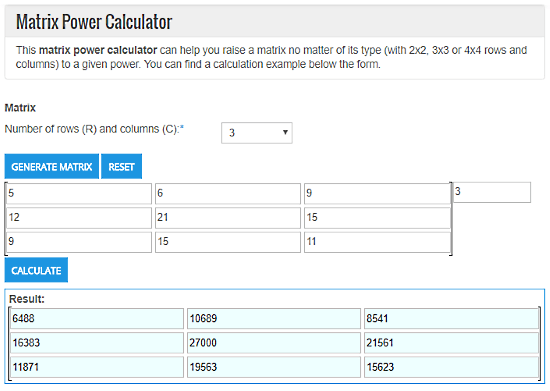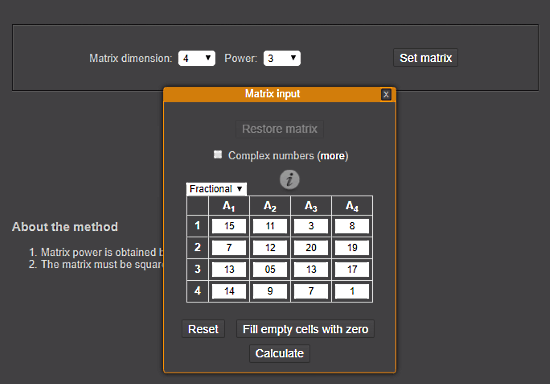Editor Ratings:
User Ratings:
[Total: 0 Average: 0]

This article covers 5 free Matrix Power Calculator websites where you can calculate the power of any given square matrix.

With these online calculators, you can raise the power of any possible square matrix and find its solution. You can only generate square matrices (equal number of rows and columns) in these calculators. They do not support matrices with different numbers of rows and columns. These calculators have maximum limits for matrix size and exponent value, but in most of them, the limit is still pretty high for regular calculations.

Also Read: 5 Best Free Online LaTex Matrix Generator Websites

## TheCalculator.coTheCalculator.co is an online calculator with all kinds of calculation tools. With its Matrix Power Calculator tool, you can easily calculate the power of a square matrix. To do so, first, generate a matrix by selecting the number of rows and columns, and fill up the values.

This calculator supports negative and decimal values along with whole numbers. You can generate 1×1 to 4×4 matrices here. Then, enter the exponent value (power) which you want to calculate for that matrix in the exponent box at the top right corner of the matrix. After that, click the ‘Calculate‘ button to get the resulted matrix.

Give this Matrix Power Calculator a try here.

## OnlineMSchool.comOnlineMSchool.com is designed to help out user with mathematical queries and calculations. It has a Matrix Power Calculator where you can enter your matrix values and calculate its power. You can calculate the power of any square matrix with matrix size from 2×2 to 7×7. You can use all integers, decimal, and fraction values in the matrix but the exponent must be a positive integer in between 1 and 100.

This Matrix Power Calculator shows you the step by step calculations. You can also see the further detailed calculations as well.

Try OnlineMSchool‘s Matrix Power Calculator here.

## MatrixReshish.comMatrixReshish.com‘s Matrix Calculator is a simple tool to calculate matrix power. Along with fraction and decimal values, this calculator also supports complex numbers. Here, you can generate a square matrix with dimensions from 2 to 100. Same range is supported by the matrix power as well. As the dimension range is quite big for a matrix, it has a button which is labeled as ‘Fill empty cells with zero‘. In case of big matrices, you can enter the non-zero values only and fill the remaining cells with zeros by this button.

This matrix calculator shows you step by step calculation. It also gives you an option to multiple the resulted matrix by another matrix, which will be convenient if you have to perform further calculations.

Calculate Matrix Power with MatrixReshish here.

## Calculator.vhex.netCalculator.vhex.net is another online calculator where you can perform various mathematical calculations tasks like Statistics, Linear Algebra, Trigonometry, Polynomials, etc. It offers an Integer power of a square matrix calculator tool to calculate matrix power. This tool support square matrix of 3×3 to 9×9 size. Here, you can calculate matrix power for exponent value in between 1 and 20.

The main attraction of this tool is its batch operation mode. Here, you can upload an Excel or CSV file for batch operation, and it will calculate the power for all those matrices which are under its limits.

Update 2022: This website doesn’t exist anymore. We recommend you use Keisan Online Calculator instead.

## Matrixcalc.orgMatrixcalc.org is an online matrix calculator that supports almost every matrix operation. You can use any possible integer, decimal, and negative value in the matrix here. There is no maximum matrix size and power limit mentioned here. To test that, I raised the power of a 20×20 matrix by 999 and it did give me the solution for that.

To calculate matrix power here, generate a desired square matrix layout and fill up the value in either of the matrix (Matrix A or Matrix B). Then enter the desired exponent value beside ‘Raise to the power of‘ button below that matrix. After that, click that button to calculate the power.

You can try this Matrix Power Calculator here.

### Final Verdict:

All these Matrix Power Calculator websites are easy to work with. You can use any of them for regular matrix power calculations. And, if you are dealing with non-regular calculations then your options get limited. In that case, Matrixcalc.org should work for you.

 Editor Ratings: User Ratings:[Total: 0 Average: 0] Tags: Matrix calculator